•研究论文
•研究论文
• 为了进一步提高极限学习机的学习性能，将并行学习的思想引入单层极限学习机，并提出了基于并行学习的多层极限学习机模型。实验结果表明，该模型比传统的单层极限学习机、多层极限学习机以及传统基于误差反向学习的...神经网络 稀疏编码
• 对ELM从命名开始说起，一直到公式原理的介绍一步步的带领掌握ELM.人工智能
• 基于改进的多层极限学习机和小波变换的高炉渗透率预测模型研究论文
• 极限学习机matlab程序。程序里包括样本训练、测试、精度、隐层神经元个数、激活函数选取。程序
• 为了快速而准确地判别煤矿突水水源类型，提出了一种构建多层正则极限学习机(M-RELM)模型的方法，该模型融合了非线性特征提取和分类学习。以激光诱导荧光(LIF)技术获取水样荧光光谱，作为模型的输入；以改进的自动...
• 基于极限学习机的自编码器(ELM-AE) 文章目录基于极限学习机的自编码器(ELM-AE)1.算法原理2.算法实验2.1 利用ELM-AE对单维数据进行自编码2.2 利用ELM-AE对图像进行编码与还原3.参考文献4.Matlab代码 1.算法原理 ELM...
基于极限学习机的自编码器(ELM-AE)

文章目录
基于极限学习机的自编码器(ELM-AE)1.算法原理2.算法实验2.1 利用ELM-AE对单维数据进行自编码2.2 利用ELM-AE对图像进行编码与还原
3.参考文献4.Matlab代码

1.算法原理
ELM基础原理请参考：https://blog.csdn.net/u011835903/article/details/111073635。
自动编码器 AE（Auto Encoder）经过训练可以将输入复制到输出。因为不需要标记数据，训练自动编码器是不受监督的。因此，将AE的思想应用到ELM中，使ELM的输入数据同样被用于输出，即输出Y=X。作为自编码器的极限学习机ELM-AE网络结构如图1所示。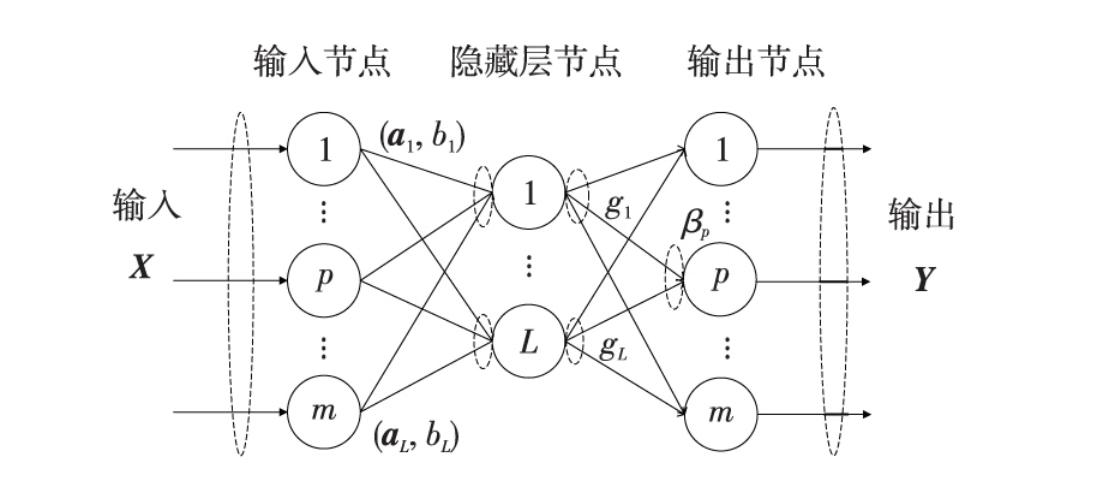图1.ELM-AE网络结构图

若图1中m>L ，ELM-AE实现维度压缩，将高维度数据映射成低维度特征表达；若 m=L，ELM-AE实现等维度的特征表达；若 m<L ,ELM-AE实现稀疏表达，即原始数据的高维特征表达。
综上，ELM-AE是一个通用的逼近器，特点就是使网络的输出与输入相同，而且隐藏层的输入参数

(

a

i

,

b

i

)

(a_i,b_i)

​​随机生成后正交。正交化后的优点有：
（1）根 据 J-L(Johnson-Lindensrauss) 定理，权重和偏置正交化可以将输入数据映射到不同或等维度的空间，从而实现不同功能的特征表达。
（2）权重和偏置的正交化设计可以去除特征以外的噪声，使特征之间均匀，且更加线性独立进而增强系统的泛化能力。
ELM-AE的输出可以用如下表达式表示：

x

j

=

∑

i

=

1

L

β

i

G

(

a

i

,

b

i

,

x

j

)

,

a

i

∈

R

m

,

β

i

∈

R

m

,

j

=

1

,

2

,

.

.

.

,

N

,

a

T

a

=

I

,

b

T

b

=

1

(1)

x_j=\sum_{i=1}^L \beta_iG(a_i,b_i,x_j),a_i\in R^m,\beta_i\in R^m,j=1,2,...,N,a^Ta=I,b^Tb=1 \tag{1}

其中

a

a

是

a

i

a_i

组成的矩阵，

b

b

是

b

i

b_i

​组成的向量。隐藏层的输出权重为：

β

=

(

H

T

H

)

−

1

H

T

X

\beta = (H^TH)^{-1}HTX

其中，

X

=

[

x

1

,

.

.

.

,

x

N

]

X=[x_1,...,x_N]

是输入数据。
2.算法实验
2.1 利用ELM-AE对单维数据进行自编码
生成模拟数据：
data = sin(0:0.1:3.14);
data = data + data.^2 - data.^3;


设置ELM-AE参数如下：
ActiveF = 'sig';%设置激活函数
number_neurons = 2;%节点数

算法结果：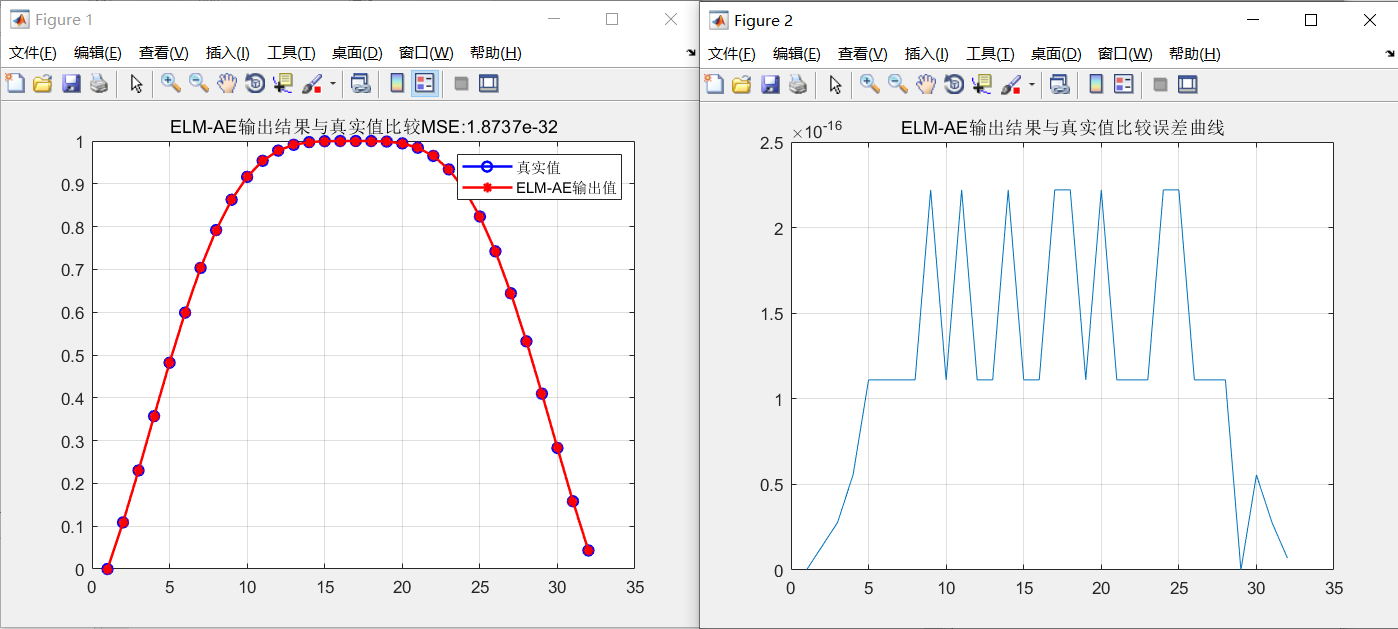从结果来看，ELM-AE输出的数据的确跟原始数据非常接近。
2.2 利用ELM-AE对图像进行编码与还原
实验对象为经典cameraman图像，利用ELM-AE设置不同的节点数，观察经过网络后，得到图像与原始图像的差异。设置结点数范围为1-50。数据输入维度为：256x256。
设置不同的结点数的结果如下：
节点数为1时：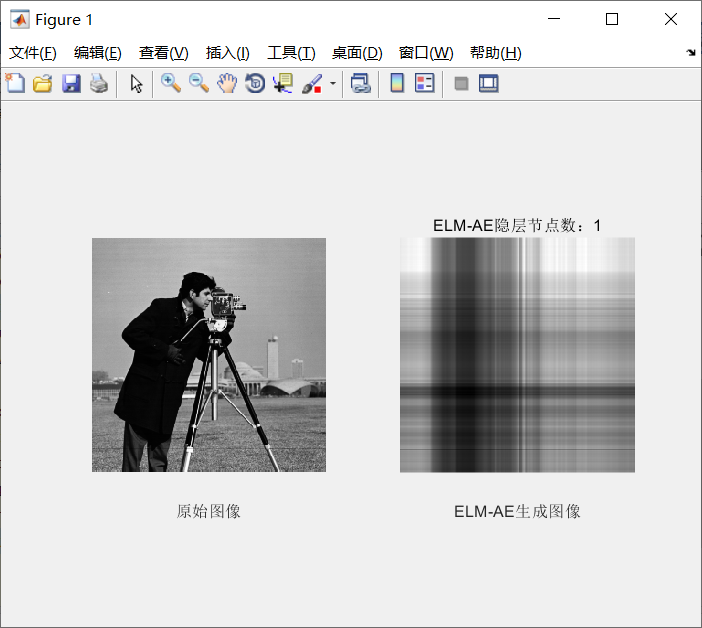节点数为10时：节点数为20时：节点数为50时：可以看到随着隐藏层节点数的增加，输出图像越来越逼近原始图像，利用仅仅50个节点，就可以使得图像跟原始图像非常接近，原始图像的维度为256，也就是说利用ELM-AE降维到50维时，编码还原后仍然能与原始图像接近。
3.参考文献
颜学龙,马润平.基于深度极限学习机的模拟电路故障诊断[J].计算机工程与科学,2019,41(11):1911-1918.
4.Matlab代码
展开全文算法 python 人工智能
• 堆叠式自动编码器和基于极限学习机的电力负荷预测混合模型研究论文
•研究论文
•研究论文
• ## 极限学习机(ELM)

千次阅读 2016-11-10 20:21:41
1. 引言极限学习机(extreme learning machine)ELM是一种简单易用、有效的单隐层前馈神经网络SLFNs学习算法。2004年由南洋理工大学黄广斌副教授提出。传统的神经网络学习算法（如BP算法）需要人为设置大量的网络训练...
1. 引言
极限学习机(extreme learning machine)ELM是一种简单易用、有效的单隐层前馈神经网络SLFNs学习算法。2004年由南洋理工大学黄广斌副教授提出。传统的神经网络学习算法（如BP算法）需要人为设置大量的网络训练参数，并且很容易产生局部最优解。极限学习机只需要设置网络的隐层节点个数，在算法执行过程中不需要调整网络的输入权值以及隐元的偏置，并且产生唯一的最优解，因此具有学习速度快且泛化性能好的优点。
其主要思想是：输入层与隐藏层之间的权值参数，以及隐藏层上的偏置向量参数是 once for all 的（不需要像其他基于梯度的学习算法一样通过迭代反复调整刷新），求解很直接，只需求解一个最小范数最小二乘问题（最终化归成求解一个矩阵的 Moore Penrose广义逆问题）。因此，该算法具有训练参数少、速度非常快等优点。接下来的若干年，黄教授带领的团队在此基础上又做了更多的发展，例如，将ELM推广到复数域，提出基于 ELM 的在线时序算法等等。
2. 基本描述
对于一个单隐层前馈神经网络(Single-hidden Layer Feedforward Networks, SLFNs)，结构如下图所示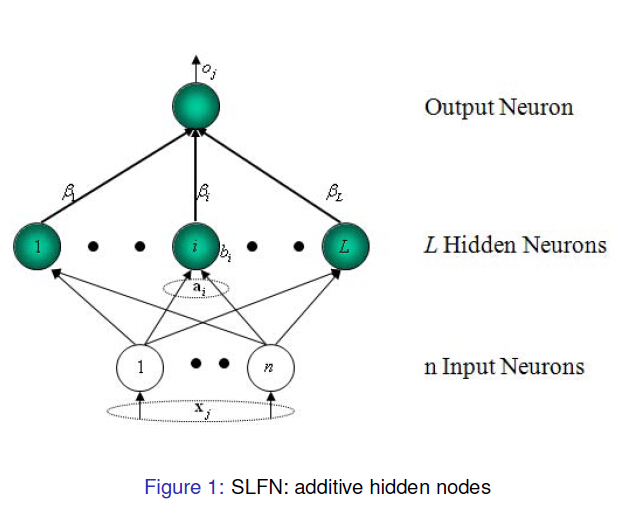为描述方便，引入以下记号：  -

N
$N$:训练样本总数
- N~$\tilde N$:隐藏层单元（以下简称隐单元）的个数  -

n,m
$n,m$:输入和输出层的维度（即输入和输出向量的长度）  -

(xj,tj),j=1,2,...,N
$(x_j,t_j),j=1,2,...,N$:训练样本（要求它们是distinct的），其中

xj=(xj1,xj2,...,xjn)T∈Rn

x_j=(x_{j1},x_{j2},...,x_{jn})^T \in R^n

tj=(tj1,tj2,...,tjn)T∈Rm

t_j=(t_{j1},t_{j2},...,t_{jn})^T \in R^m
将所有输出向量按行拼接起来，可得到整体输出矩阵

T=⎡⎣⎢⎢⎢⎢⎢tT1tT2⋮tTN⎤⎦⎥⎥⎥⎥⎥N~×m=⎡⎣⎢⎢⎢⎢⎢t11t21⋮tN1t12t22⋮tN2⋯⋯⋱⋯t1mt2m⋮tNm⎤⎦⎥⎥⎥⎥⎥

T =  \left[
\begin{matrix}
t_1^T  \\
t_2^T  \\
\vdots \\
t_N^T
\end{matrix}
\right] _{\tilde N \times m}
=
\left[
\begin{matrix}
t_{11} & t_{12} & \cdots & t_{1m} \\
t_{21} & t_{22} & \cdots & t_{2m}  \\
\vdots & \vdots & \ddots & \vdots \\
t_{N1} & t_{N2} & \cdots & t_{Nm}
\end{matrix}
\right]

-

oj,j=1,2,...,N
$o_j,j=1,2,...,N$:与标注

tj
$t_j$对应的实际输出
-

W=(wij)N~×n
$W=(w_{ij})_{\tilde N \times n}$:输入层与隐藏层之间的劝矩阵，其中

W
$W$对应第·￥i行对应的向量
wi=(wi1,wi2,...,win)T
w_i = (w_{i1},w_{i2},...,w_{in})^T

表示连接隐藏层第

i
$i$个单元与输入单元的权向量。

b=(b1,b2,...,bN~)T$b=(b_1,b_2,...,b_{\tilde N})^T$:偏置向量，

bi
$b_i$表示第

i
$i$个隐单元的阈值
β=(βij)N~×m$\beta = (\beta_{ij})_{\tilde N \times m}$:隐藏层与输出层之间的权矩阵，其中

β
$\beta$的第

i
$i$行对应的向量
βi=(βi1,βi2,...,βim)T
\beta_i = (\beta_{i1},\beta_{i2},...,\beta_{im})^T

表示连接隐藏层第

i
$i$个单元和输出单元的权向量，矩阵展开可以写成
β=⎡⎣⎢⎢⎢⎢⎢β11β21⋮βN~1β12β22⋮βN~2⋯⋯⋱⋯β1mβ2m⋮βN~m⎤⎦⎥⎥⎥⎥⎥
\beta =
\left[
\begin{matrix}
\beta_{11} & \beta_{12} & \cdots & \beta_{1m} \\
\beta_{21} & \beta_{22} & \cdots & \beta_{2m}  \\
\vdots & \vdots & \ddots & \vdots \\
\beta_{\tilde N1} & \beta_{\tilde N2} & \cdots & \beta_{\tilde Nm}
\end{matrix}
\right]

g(x)
$g(x)$:激活函数3. 模型
数学上，标准的SLFNs模型为

∑i=1N~g(wi⋅xj+bi)βi=oj,j=1,2,...,N

\sum_{i=1}^{\tilde N}g(w_i \cdot x_j+b_i) \beta_i=o_j,j=1,2,...,N

单隐层神经网络的最终目的是为了是输出的误差最小，在极限情况下为零误差逼近，可以表示为

∑j=1N||oj−tj||=0

\sum_{j=1}^N||o_j-t_j||=0

即存在

βi,wi.bi
$\beta_i,w_i.b_i$使得

∑t=1N~g(wi⋅xj+bi)βi=tj,j=1,2,...,N

\sum_{t=1}^{\tilde N}g(w_i \cdot x_j+b_i) \beta_i=t_j,j=1,2,...,N

以上N个线性方程组可以简单用矩阵表示

Hβ=T

H\beta = T
其中

H(wi,...,wN~,b1,...,bN~,x1,...,xN)

H(w_i,...,w_{\tilde N},b_1,...,b_{\tilde N},x_1,...,x_N)

=⎡⎣⎢⎢⎢g(w1⋅x1+b1)⋮g(w1⋅xN+b1)⋯⋱⋯g(wN~⋅x1+bN~)⋮g(wN~⋅xN+bN~)⎤⎦⎥⎥⎥N×N~

=
\left[
\begin{matrix}
g(w_1 \cdot x_1+b_1) & \cdots & g(w_{\tilde N} \cdot x_1+b_{\tilde N}) \\
\vdots & \ddots & \vdots \\
g(w_1 \cdot x_N+b_1) & \cdots & g(w_{\tilde N} \cdot x_N+b_{\tilde N})
\end{matrix}
\right] _{N \times \tilde N}

β=⎡⎣⎢⎢⎢βT1⋮βTN~⎤⎦⎥⎥⎥N~×N

\beta =
\left[
\begin{matrix}
\beta_1^T \\
\vdots \\
\beta_{\tilde N}^T
\end{matrix}
\right] _{\tilde N \times N}

and

and

T=⎡⎣⎢⎢TT1⋮TTN⎤⎦⎥⎥N×m

T=  \left[
\begin{matrix}
T_1^T \\
\vdots \\
T_N^T
\end{matrix}
\right] _{ N \times m}

为了能够训练单隐层神经网络，我们希望得到

βi,wi.bi
$\beta_i,w_i.b_i$，使得

||H(w^1,...,w^N~,b^1,...,b^N~)β^−T||=minw,b,β||H(w1,...,wN~,b1,...,bN~)β−T||

||H(\hat w_1,...,\hat w_{\tilde N},\hat b_1,...,\hat b_{\tilde N})\hat \beta-T||= \min_{w,b,\beta}||H(w_1,...,w_{\tilde N}, b_1,...,b_{\tilde N})\beta-T||

其最小二乘解便为

β^=H+T

\hat \beta = H^+T

其中

H+
$H^+$是

H
$H$的广义逆矩阵，利用SVD分解等许多方法均可以求出。

传统方法是写出目标函数，然后求解梯度，利用BP原理算法反向传播误差，BP算法存在一些缺点：
1. 学习率的取值。学习率过小，则收敛非常慢；学习率过大，则算法不稳定，可能会发散。
2. 局部最优。若损失函数不是凸函数，则算法可能陷入局部最优解。
3. 过拟合。BP算法如果参数设置不当，则可能会造成过拟合，使得泛化能力变差，因此需要引入交叉验证和极小化过程中引入合适的停止机制。
4. 耗时。显然基于梯度的学习算法通常都比较耗时。

相比较BP算法，ELM算法最大的优势便是速度极快，当然准确率有可能会损失一些。另外基于梯度求解的算法就是有可能陷入到局部最优解出不来，而ELM是基于最小二乘原理所以不存在这个。当然ELM算法也存在缺点，就是单层限制，在多层的情况下不像BP算法那样梯度存在链式法则很好处理。

4. 算法步骤

任意赋值输入权值wi,bi,i=1,2,...,N~$w_i,b_i,i=1,2,...,\tilde N$
计算隐层输出矩阵

H
$H$
计算输出权矩阵β^=H+T$\hat \beta = H^+T$
展开全文神经网络 算法
• 具有基于随机稀疏矩阵的自动编码器的增强型分层极限学习机研究论文
• 深度极限学习机(DELM)目前主要含两种基本结构：1、利用传统深度学习框架(主要是堆栈自动编码器，或者是深度置信网络)进行数据的深度自适应特征提取，而后将获得的特征输入至极限学习机及其变种中进行分类或回归；...
       深度极限学习机(DELM)目前主要含两种基本结构：1、利用传统深度学习框架(主要是堆栈自动编码器，或者是深度置信网络)进行数据的深度自适应特征提取，而后将获得的特征输入至极限学习机及其变种中进行分类或回归；2、利用极限学习机与自动编码器相结合，形成极限学习机-自动编码器(ELM-AE)，结构如图1，将ELM-AE作为无监督学习的基础单元对输入数据进行贪婪逐层无监督预训练，并保存ELM-AE由最小二乘法获得的输出权值矩阵，然后用这些输出层权重来初始化多层极限学习机(ML-ELM，也称深度极限学习机，Deep extreme leaning machine，DELM)，最后结合标签信息对DELM的输出层权重进行学习，结果图2所示。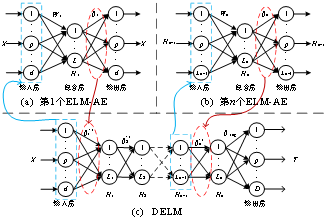关于第一种深度极限学习机，可改进的地方较少，大部分人就是将不同的深度学习方法与不同的极限学习机变种进行结合，方法较为简单。我们今天就说说第二种深度极限学习机，后文所述的深度极限学习机默认是第二种。
和其他深度学习相比，深度极限学习机无需微调，ELM-AE与最终的DELM分类层(或回归层)均采用最小二乘法且只进行一步反向计算得到更新后的权重，因此显著特点是速度快。缺点也很明显，第一，ELM-AE的输入层权值与偏置依旧是随机生成，且不进行反向调整，因此DELM的精度ELM-AE输入权值的影响；第二，采用多隐含层结构的DELM，各层节点数对最终精度有着较大影响；第三，采用最小二乘法进行输出权值一步更新，在样本数较少时比较方便，当处理大样本问题时（比如MNIST），在计算Moore伪逆的时候极大地增加了计算时间与复杂度，并且特别容易出现欠拟合。
今天，我们针对问题2进行解决，利用粒子群对各ELM-AE的输出层权值与偏置进行优化，简称PSO-DELM，并用这个算法进行轴承故障诊断。
1.数据准备
采用凯斯西储轴承故障诊断数据，驱动端，48k采样频率，0HP负载，共10类数据，如下所示。从每类数据中各取100个样本，每个样本长度为864个采样点，共得到1000个样本，然后按照7:3划分训练集与测试集。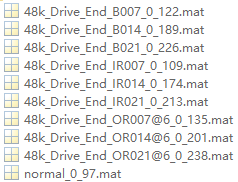2.数据预处理
对上述采集的样本进行FFT特征提取，提取每个样本的频谱幅值作为最终的特征数据，如下图所示。然后归一化，作为DELM与PSO-DELM的输入数据，标签采用onehot编码，即第一类为[1 0 0 0 0 0 0 0 0 0]，第二类为[0 1 0 0 0 0 0 0 0 0]。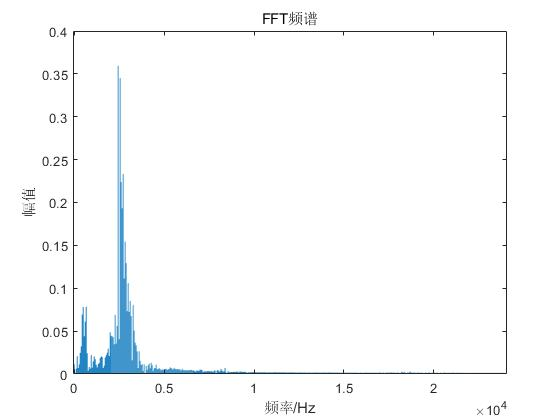3.DELM故障诊断分类
首先利用ELM-AE对2中数据集进行逐层无监督预训练，保存各ELM-AE的输出层权重，然后用上述权重初始化DELM，有点类似于堆栈自动编码器。最后结合标签数据进行DELM分类层的训练，ELM-AE与DELM均采用最小二乘法进行。测试集结果如下：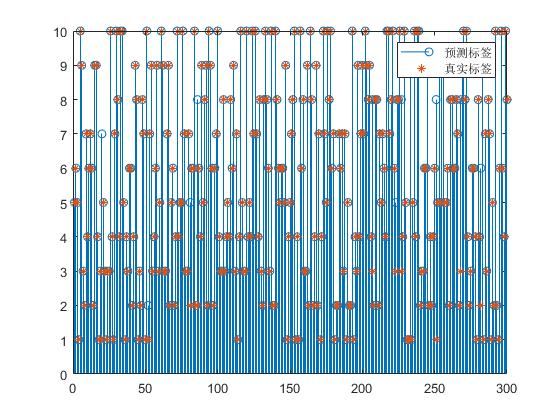诊断精度为96.67%
4.PSO-DELM故障诊断分类
以最小化分类错误率作为适应度函数，PSO算法的目的是找到一组ELM-AE的初始权重使得DELM的错误率最低，因此适应度进化曲线是一条下降的曲线，表示随着pso优化的，找到更好的初始权重，使得delm的分类错误率越来越低。结果如下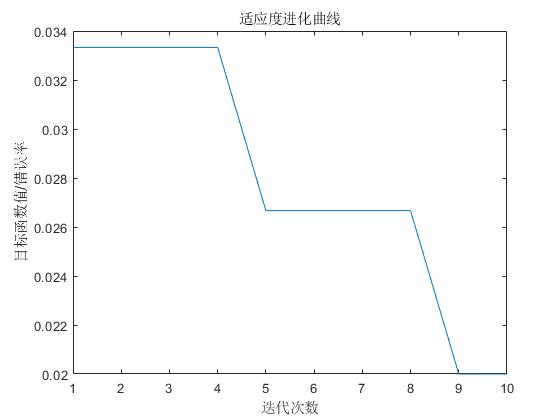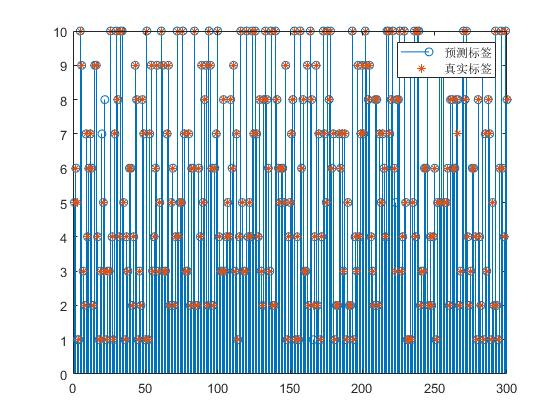诊断精度为98%。
5.结论
针对DELM中各ELM-AE采用随机初始权值与偏置的问题，采用粒子群算法对其这些ELM-AE的初始权重进行优化，结果表明，优化的DELM具有更好的诊断精度
代码：点这里


展开全文人工智能 算法 神经网络
•研究论文
• 一开始，ELM 建议用于训练（SLFN）单隐藏层前馈神经网络，似乎能够扩展到 MLP（多层感知器）。 在这段代码中，我们提出了一种基于 ELM 的算法来训练用于回归和分类的 MLP。matlab
• 在上一篇《DeepLearning 的挑战： Extreme Learning Machine（超限学习机）？》 中介绍了一些ELM与DL 的一些比较，这篇主要介绍ELM的原理。 首先，ELM的核心就是将复杂的迭代过程转变为隐层参数随机产生。 其次，ELM...
在上一篇《DeepLearning 的挑战： Extreme Learning Machine（超限学习机）？》 中介绍了一些ELM与DL 的一些比较，这篇主要介绍ELM的原理。
首先，ELM的核心就是将复杂的迭代过程转变为隐层参数随机产生。
其次，ELM 是一个神经网络，有输入层、隐藏层，输出层。
最后，ELM 的多分类效果优于SVM，而且速度贼快。

对于训练样本集{xi,ti}  i=1-N, 共有N各样本，其中每个样本xi 是一个d维列向量，ti是输出标签。
ELM,的输出为：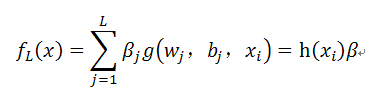其中，wj 为连接第j 各隐节点的输入权值；bj 为第j个隐节点的偏差； j 为第j各隐节点到ELM输出节点的权值；g（）为第j各隐节点的输出函数；
而， h（xi）=[g(w1,b1,xi),…..,g(wL,bL,xi)]为隐层关于xi的输出向量，h（xi）的作用是将xi由d维空间映射到L维特征空间。
优化：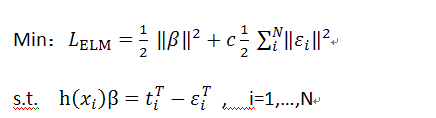其中，   是训练误差，消除过拟合，从而减少测试误差。
C为惩罚因子。
类似于SVM，用对偶问题求解：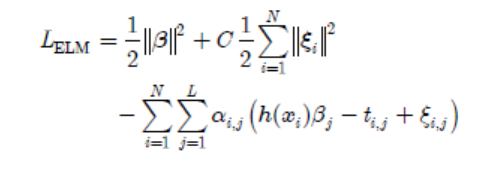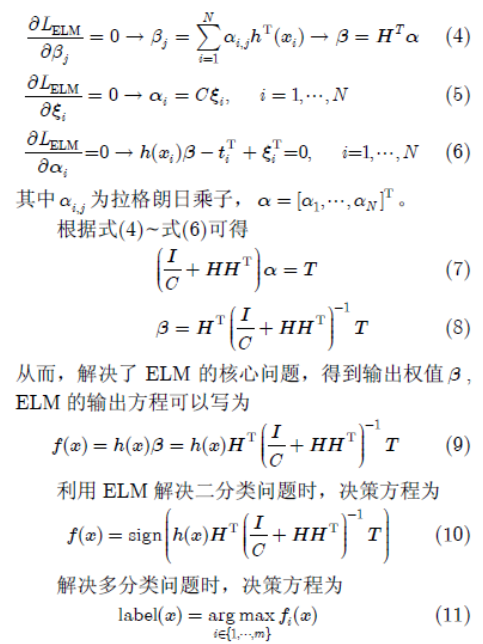公式相对于SVM来说，已经很少了。其中，随机化隐层参数a，b，无需迭代求解。
个人觉得，其模拟人脑系统确实更实际，如果人脑像迭代似的，最优化参数，那速度也贼慢。反而，我们用不同g() 隐层函数，如线性函数，sign函数，甚至cos，sin函数等等，这些学习到的特征更多，而且我们如果将这些函数深层网络格式，这样，是不是又能组合出不一样的函数呢？
所以，我觉得，随机化这些参数是有意义的，而且，效果不错，就能证明他有实用空间。
以下是截图：更能清楚看到SVM和ELM的关系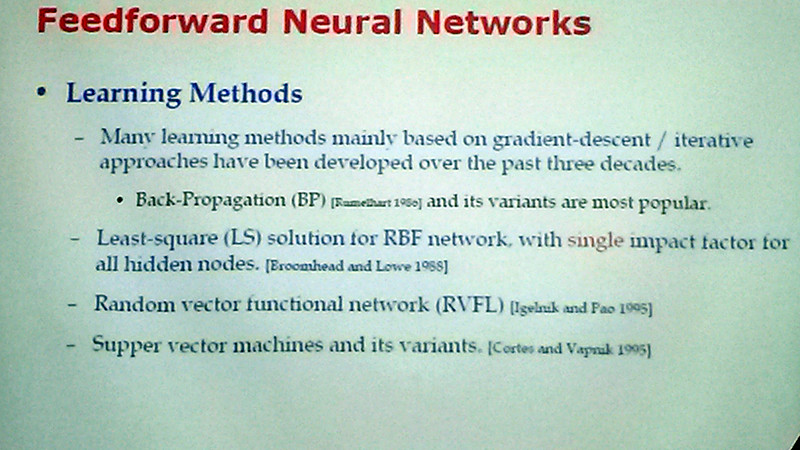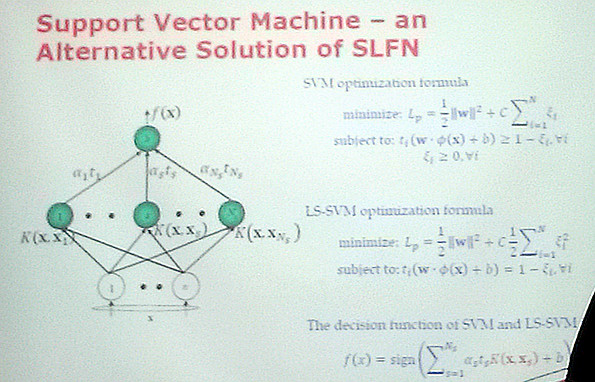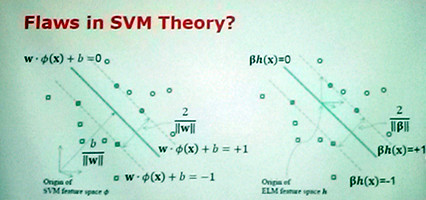ps：CSDN 的公式编辑贼麻烦！

参考：
  融合ELM学习机
  peghoty 的ELM博文 （简要介绍了SLFN）
 ELM (extreme learning machine) 官方 （资料就很全了！）
展开全文ELM svm 深度学习
• 8非线性规划BP神经网络基于PSO工具箱的函数优化支持向量机分类极限学习机回归拟合及分类基于多层编码遗传算法的车间调度
• 基于深度极限学习机DELM的分类 文章目录基于深度极限学习机DELM的分类1.ELM原理2.深度极限学习机（DELM）原理3.实验结果3.参考文献4.Matlab代码 1.ELM原理 ELM基础原理请参考：...神经网络 人工智能 算法
• 深度极限学习机也叫多层极限学习机ML-ELM，其首先采用多个极限学习机-自动编码器(ELM-AE)进行无监督预训练，然后利用各ELM-AE的输出权重用于初始化整个DELM。相比于其他深度方法，DELM具有训练速度快的优点，但是...时间序列预测
• 用于R语言的多层感知器（MLP）和极限学习机（ELM）进行时间序列预测。请注意，由于神经网络无法利用GPU处理，因此大型网络的训练速度往往很慢。与现有神经网络实现的不同之处在于，R可以自动设计具有合理预测性能的...
• 针对传统深度核极限学习机网络仅利用端层特征进行分类导致特征不全面，以及故障诊断分类器中核函数选择不恰当等问题，提出基于多层特征表达和多核极限学习机的船舶柴油机故障诊断方法。利用深度极限学习机网络提取...
• 用于极限学习机的高性能工具箱。 极限学习机（ELM）是一种特殊的人工神经网络，可以解决分类和回归问题。 它们的性能可与使用错误反向传播算法训练的经典多层感知器相媲美，但训练时间最多可缩短6个数量级。 （是，...
• 本模型是基于极限学习机和遗传算法两种的优化和组合，并且得到了总合85%的预测成果。ELM和GA两模型的介绍如下： 极限学习机（Extreme LearningMachine,ELM）或“超限学习机”是一类基于前馈神经网络（Feedforward ...神经网络 python 数据挖掘
• 对于此示例，我将对AirPassengersR中可用的时间序列进行建模。我将最后24个观察值保留为测试集，并将使用其余的观察值来拟合神经网络。当前有两种类型的神经网络可用，多层感知器；和极限学习机R语言 神经网络 时间序列
• 摘要：ELM算法执行过程中不必调整网络的输入权值以及隐元的偏置，学习速度快且泛化...【编者按】被认为学习速度快、泛化性能好的Extreme Learning Machine（ELM，极限学习机），在国内颇有市场，但大神Yann LeCun近日
• 极限学习机和支持向量机 了解机器学习 (Understanding ML) Note! This is just a major overview of the ELM evolution. It doesn't include all possible versions and tweaks done to ELMs through the years. ...python 人工智能
• 定义极限学习机器( Extreme Learning Machine，ELM) 是神经网络研究中的一种算法，是一种泛化的单隐层前馈神经网络( Single-hidden Layer Feed forward Network，SLFN)［1］。特点输入权值和隐层阈值进行随机赋值，......